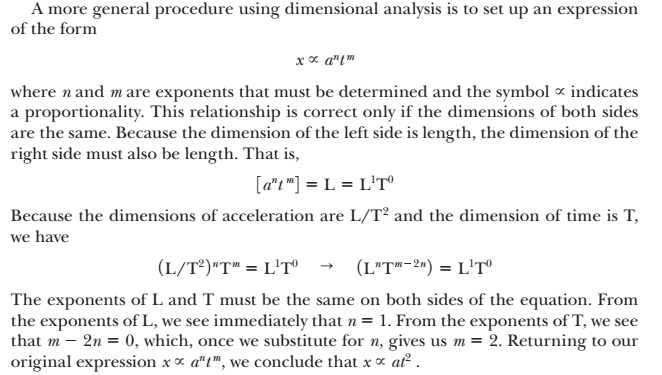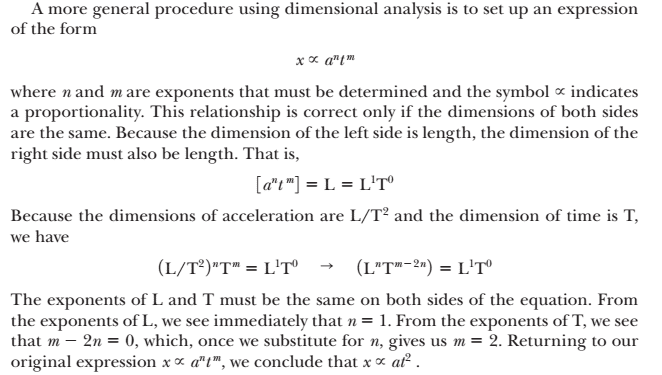# A question on Dimensional analysis

This is actually an example from Physics for Scientists and Engineers by Serway. I am confused about the way they solved it.

## Homework Statement

Suppose we are told that acceleration a of a particle moving with uniform speed v in a circle of radius r is proportional to some power of r, say r^m, and some power of v, say v^m. Determine the values of n and m and write the simplest form of an equation for the acceleration.

## Homework Equations

The problem is solved in the book. But I dont understand the solution properly.This problem was solved in the example as below:

$$a = k(r^n)(v^m)$$$$L/T^2 = (L^n)(L/T)^m = L^{n+m}/T^m$$$$n+m = 1$$$$m = 2$$$$n = -1$$$$a = kr^{-1}v^2$$

## The Attempt at a Solution

From the question we don't know if k has any dimension or not. So how did they (in the second line) write $$L/T^2=(L^n)(L/T)^m ?$$ Where did the k go??

Nathanael
Homework Helper
k is by definition dimensionless. It's the constant of proportionality (meaning it's a constant that changes it from being "proportional" to being "equal")

The definition of "a" being proportional to "b" is a=kb where k is some constant (hence it's a pure, dimensionless, number)

Last edited:
OOPPss... Suddenly I understand. k is not anything like length mass or time, right? Maybe thats the reason why they left out k?

Wait, I have another question. In law of gravitation we know F = GmM/r^2 Here G is a proportionality constant, isn't it? Then how come it is not a pure number (ie it has units like) 6.67×10^−11 N·(m/kg)2 ?

Last edited by a moderator:
Nathanael
Homework Helper
OOPPss... Suddenly I understand. k is not anything like length mass or time, right? Maybe thats the reason why they left out k?

Correct it's a unitless number. (I think such a number is ofted called a "pure number")

EDIT:
Wait, I have another question. In law of gravitation we know F = GmM/r^2 Here G is a proportionality constant, isn't it? Then how come it is not a pure number (ie it has units like) 6.67×10^−11 N·(m/kg)2 ?

Good question. I don't know a satisfactory answer. It seems I was wrong when I said "k is by definition dimensionless," I suppose it doesn't necessarily have to be dimensionless.

(Which of course brings us back to your original question, how do we know k is dimensionless in your original post?)

Sorry about the misinformation, I'm not sure why it must be dimensionless in your OP.

Last edited:
Nathanael
Homework Helper
Perhaps it's because they asked for "the simplest form of an equation for the acceleration."

(Or perhaps it's just to avoid the infinite possibility that comes with k being of choose-able units)

Perhaps it's because they asked for "the simplest form of an equation for the acceleration."

It never seems to be the case. Serway's book describes it as a general procedure. Here is the excerpt from Serway's book:(as this page can be previewed in amazon; I think it never breaks any copyright)Now this certainly works for x ∞ (a^n)(t^m); but what happens when we apply this for, say - the law of gravitation? Here, F ∞ Mm/r^2 certainly doesn't pass the test of dimensional analysis (?). I am confused!

SteamKing
Staff Emeritus
Homework Helper
It never seems to be the case. Serway's book describes it as a general procedure. Here is the excerpt from Serway's book:(as this page can be previewed in amazon; I think it never breaks any copyright)Now this certainly works for x ∞ (a^n)(t^m); but what happens when we apply this for, say - the law of gravitation? Here, F ∞ Mm/r^2 certainly doesn't pass the test of dimensional analysis (?). I am confused!

Well, why don't you apply dimensional analysis to the law of gravitation? Hint: not every constant of proportionality is unitless, like the π in A = π r$^{2}$ for the area of a circle. And besides, 'constant' means the value doesn't change; it doesn't mean there are no units.

•1 person
Thanks.
So I conclude - dimensional analysis works for equations; but it will work for proportionality only if the constant is unit less. (The book was a bit confusing.)